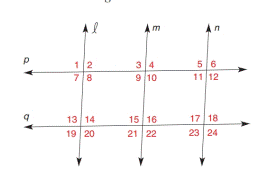Chapter 2.3, Problem 12EElementary Geometry For College St...

7th Edition
Alexander + 2 others
ISBN: 9781337614085

Solutions

Chapter
SectionElementary Geometry For College St...

7th Edition
Alexander + 2 others
ISBN: 9781337614085
Textbook Problem

In Exercise 7 to 16, name the lines (if any) that must be parallel under the given conditions.l ∥ m and m ∥ n

To determine

To find:

The parallel lines.

Explanation

Given:

The given statement is,

m and mn

Approach:

If two lines are each perpendicular to a third line, then these lines are parallel to each other.

Calculation:

The given statement is,

m and mn

Still sussing out bartleby?

Check out a sample textbook solution.

See a sample solution

The Solution to Your Study Problems

Bartleby provides explanations to thousands of textbook problems written by our experts, many with advanced degrees!

Get Started

In problems 27-30, find. 28.

Mathematical Applications for the Management, Life, and Social Sciences

Solve the equations in Exercises 126. 6x(x2+1)2(x2+2)48x(x2+1)3(x2+2)3(x2+2)8=0

Finite Mathematics and Applied Calculus (MindTap Course List)

Prove that cosine is a continuous function.

Single Variable Calculus: Early Transcendentals

What characteristic differentiates a pretest-post test design from a time-series design?

Research Methods for the Behavioral Sciences (MindTap Course List)